### IMO Shortlist 1998 problem G3

Kvaliteta:
Avg: 0,0
Težina:
Avg: 7,0
Let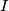$I$ be the incenter of triangle$ABC$. Let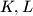$K,L$ and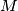$M$ be the points of tangency of the incircle of$ABC$ with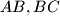$AB,BC$ and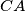$CA$, respectively. The line$t$ passes through$B$ and is parallel to$KL$. The lines$MK$ and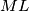$ML$ intersect$t$ at the points$R$ and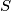$S$. Prove that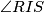$\angle RIS$ is acute.
Izvor: Međunarodna matematička olimpijada, shortlist 1998